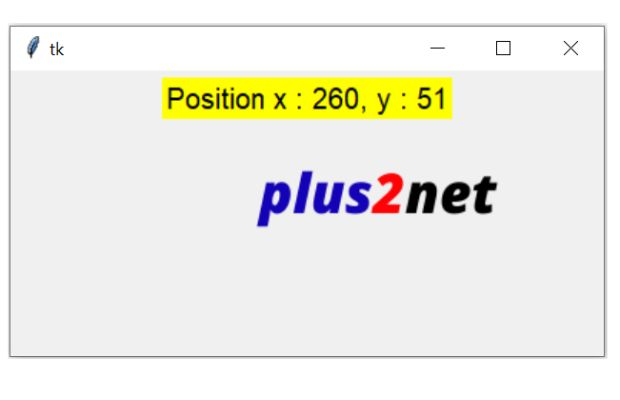# Drag and Drop Mouse Event`<B1-Motion> ` Mouse Left button Press and move

We can read the x ( horizontal ) and y ( vertical ) coordinates of the mouse pointer when left buton is pressed and mouse is in motion.

Here is the script to display x and y coordinates when mouse is in motion ( left button is pressed )

Used one Label to display the coordinates. By using config() inside a callback function my_callback() we will update the text option of the Label by displaying the changing values of x and y coordinates.

Here is the event which will trigger the callback function my_callback()
``my_w.bind('<B1-Motion>',my_callback)``
Full code is here.
``````import tkinter as tk
my_w = tk.Tk()
my_w.geometry("615x400")  # width and height
def my_callback(event):
l1.config(text='Position x : '+ str(event.x) +", y : "+ str(event.y))
l1=tk.Label(my_w,text='to Display',bg='yellow',font=30)
my_w.bind('<B1-Motion>',my_callback) # Mouse left button pressed move
my_w.mainloop()``````

Tkinter Drag and Drop image using Left mouse button press and move event using canvas

We will add one canvas to the above window and place one image inside the canvas.
``````f_img = tk.PhotoImage(file = "D:\\top2.png") # path of the image
my_c = tk.Canvas(my_w,width=600,height=400) # canvas size
my_c.pack() # place on pack
my_img = my_c.create_image(180, 50,  image=f_img) # add image to canvas``````

## Drag and Drop ( DND )

We will move the image by updating the x and y coordinates of the image ( inside the canvas ) by reading the event.x and event.y values.
``````import tkinter as tk
my_w = tk.Tk()
my_w.geometry("615x400")  # width and height
def my_callback(event):
global f_img
l1.config(text='Position x : '+ str(event.x) +", y : "+ str(event.y))
f_img = tk.PhotoImage(file = "D:\\top2.png")
my_img = my_c.create_image(event.x, event.y,  image=f_img)
l1=tk.Label(my_w,text='to Display',bg='yellow',font=30)
f_img = tk.PhotoImage(file = "D:\\top2.png") # path of the image
my_c = tk.Canvas(my_w,width=600,height=400) # canvas size
my_c.pack() # place on pack
my_img = my_c.create_image(180, 50,  image=f_img) # add image to canvas
my_w.bind('<B1-Motion>',my_callback) # Mouse left button pressed move
my_w.mainloop()``````

Subscribe to our YouTube Channel here

## Subscribe

* indicates required
Subscribe to plus2netplus2net.com